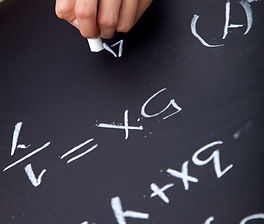top of page##### Expressways To Math®

Level 1 and A-Vip

Expressways To Math®  makes use of the same A-VIP techniques used in Expressways To Reading®. A-VIP is used to embed into permanent memory the fundamental facts of calculation. Expressways To Math®  then exercises and integrates the accumulating memory base so that the learner can apply it to developing math skills through computer games, recitation to music and rhythms, and contests.Level I simultaneously integrates number theory, counting, addition, subtraction, multiplication and division as applied to practical situations. The goal is to commit to memory the 400 math facts, to understand counting and number theory, and to be able to recall the facts for quick mental applications. When the math facts are thoroughly mastered, students often generate computational speeds that rival electronic calculators.Level 2 and Level 3

Expressways To Math®  Level II involves telling time, handling money, inequalities, types of fractions and their processes, decimal numbers, ruler fractions, percentages, measurements in the English and metric systems, basic plane geometric forms, averages, estimates, ratio, probability, exponents, circles, areas of circles, volumes of solids, negative numbers, temperature and some advanced lessons for logical thinkers. Each lesson has an interactive instructional introduction before a student is directed to perform the exercises.

Level III consists of the section of word problems from QuikComp®.

bottom of page